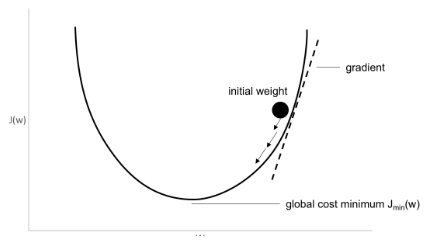The gradient descent algorithm performs multidimensional optimization. The objective is to reach the global maximum. Gradient descent is a popular optimization technique used in many machine-learning models. It is used to improve or optimize the model prediction. One implementation of gradient descent is called the stochastic gradient descent (SGD) and is becoming more popular (explained in the next section) in neural networks. Optimization involves calculating the error value and changing the weights to achieve that minimal error. The direction of finding the minimum is the negative of the gradient of the loss function. The gradient descent procedure is qualitatively shown in the following figure:The learning rate determines how big each step should be. Note that the ANN with nonlinear activations will have local minima. SGD works better in practice for optimizing non-convex cost functions.